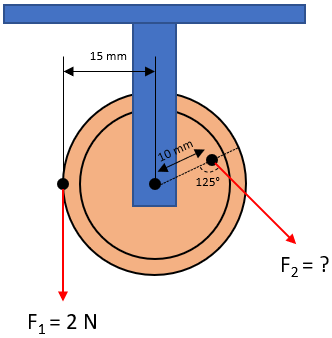## Moment of a Force

In the below figure, a freely rotating pulley is acted on by two forces. Determine the magnitude for the second force that would make the pulley be in rotational equilibrium.Hint
$$F_1=F_2$$$More specifically, the question is asking for the toques/moments to be equal. $$\tau_1=\tau_2$$$
$$\tau=F\cdot rsin(\theta)$$$where $$F$$ is the force, $$r$$ is the length of the moment arm, and $$\theta$$ is the angle between the force vector and moment arm. The question is asking for $$F_1=F_2$$$
$$\tau_1=\tau_2$$$Remember, to find torque: $$\tau=F\cdot rsin(\theta)$$$
where $$F$$ is the force, $$r$$ is the length of the moment arm, and $$\theta$$ is the angle between the force vector and moment arm.
$$\tau_1=(2N)\cdot (0.015m)sin(90^{\circ}) =(2N)(0.015m)(1)=0.03\:N\cdot m$$$Now that both torques are known, let’s solve for $$F_2$$ using the same equation: $$\tau_1=\tau_2=F_2\cdot (0.01m)sin(125^{\circ})=0.03\:N\cdot m$$$
$$F_2=\frac{0.03N\cdot m}{(0.01m)(0.819)}$$$$$F_2=\frac{0.03N\cdot m}{(0.01m)(0.819)}=\frac{0.03N\cdot m}{0.00819m}=3.66\:N$$$